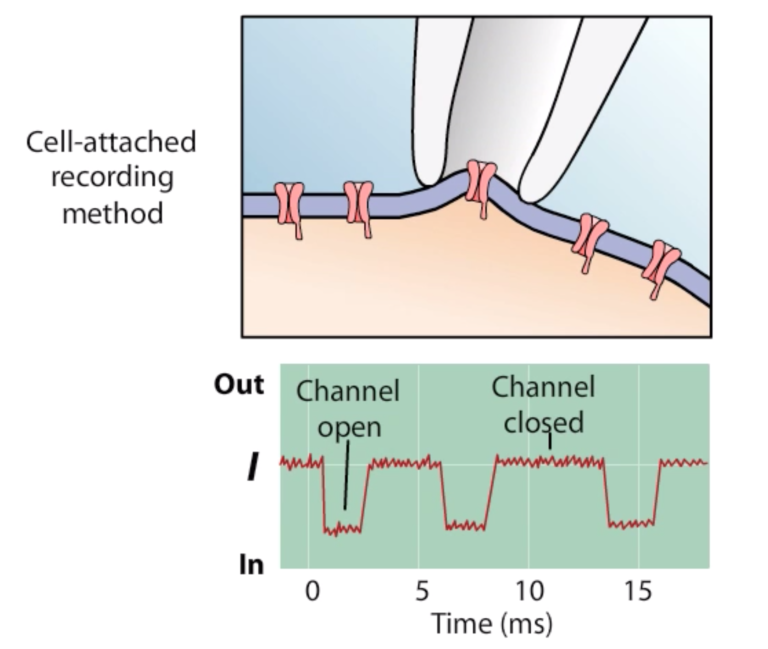# A Brief Introduction to the Patch Clamp Technique and the Hodgkin–Huxley Equations

Patricia Martínez Díaz

6. April. 2021

## Hello and welcome back!

Now that we had explained some of the basics of electrophysiological modeling in the previous posts, this time I am going to talk about the Patch clamp technique and to further explain the equations that describe the action potential of an electrical cell, known as the Hodgkin-Huxley equations.  You might recall that Hodgkin–Huxley modeled the neuron of a squid’s giant axon. They did this by using an electrical analogue circuit of the cell membrane and also by applying Ohm´s law in order to calculate the voltages and currents in the circuit.

### 1. The Patch Clamp Technique

Hodgkin–Huxley recorded the action potential from inside of a nerve cell through an in-vitro experiment. One of the most popular methods used to study ionic currents is the patch clamp technique. During this assay, a tiny glass pipette suctions the cell membrane creating a “patch” and isolates the membrane, as seen in the image below. An amplifier is connected to the pipette and allows to record the current of the patch. A nice animation explaining the patch clamp technique can be seen in the following video.Figure 1. The Patch clamp technique recording changes in ionic currents due to opening or closing of ionic channels. Obtained from: https://www.youtube.com/watch?v=mVbkSD5FHOw

### 2. An introduction to the Hodgkin-Huxley equations

Take a look at the following image:

– What do you see?

– Can you recognize all the elements in the circuit?

This is the electrical analogue circuit used to explain the voltage changes in the cell membrane caused by ionic gates kinetics and it is similar to the one proposed by Hodgkin–Huxley. It has 2 nodes, represented by the black points in the diagram, and 4 different paths arranged in parallel representing the currents of the sodium channel (INA), Potassium channel (IK), Leakage current (IL), caused by the experimental method itself, and the current through the cell membrane.

Using node analysis, it is possible to calculate the total transmembrane current (IInter), which is the sum of all the currents passing through each of the branches, as described in the following equation: